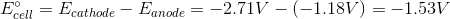# Physical Chemistry : Galvanic and Electrolytic Cells

## Example Questions

### Example Question #1 : Galvanic And Electrolytic Cells

Which of the following is true regarding an electrolytic cell?

Oxidation happens at the anode and reduction happens at the cathode

More than one of the other answer choices is true

Solid metal is plated at the anode

The entropy of the reaction is always negative

Oxidation happens at the anode and reduction happens at the cathode

Explanation:

An electrolytic cell utilizes energy to facilitate a nonspontaneous redox reaction. This is different from a galvanic cell, which releases energy from a spontaneous redox reaction. A shared characteristic of both cells is that the oxidation half-reaction always happens at the anode and the reduction half-reaction always happens at the cathode. Note that a redox reaction always has an oxidation half-reaction and a reduction half-reaction.

The entropy of the reaction is typically negative for a nonspontaneous reaction. This is not true for all cases; therefore, the entropy could be positive or negative for an electrolytic cell. Electroplating involves reduction reactions that convert metal ions into solid metal. This solid metal is coated onto a metal plate. Since this involves a reduction reaction, it occurs at the cathode.

### Example Question #1 : Galvanic And Electrolytic Cells

The Gibbs free energy of a redox reaction is __________ for a galvanic cell and is __________ for an electrolytic cell.

positive . . . positive

positive . . . negative

negative . . . negative

negative . . . positive

negative . . . positive

Explanation:

Gibbs free energy is the “free” energy available that can be used to perform useful work. A negative Gibbs free energy corresponds to a spontaneous reaction, whereas a positive Gibbs free energy corresponds to a nonspontaneous reaction. Recall that a galvanic cell involves a spontaneous reaction, whereas an electrolytic cell involves a nonspontaneous reaction; therefore, the Gibbs free energy of the reaction is negative for a galvanic cell and positive for an electrolytic cell.

### Example Question #1 : Electrochemistry

What type of energy input is required for a galvanic cell?

Galvanic cells do not require energy

Electrical energy

Both electrical and chemical energy

Chemical energy

Galvanic cells do not require energy

Explanation:

Recall that galvanic cells carry out spontaneous reactions; therefore, they do not require energy. They release free energy that can be used to do work such as powering an electrical device (like cell phones).

Electrolytic cells, on the other hand, require energy because they carry out nonspontaneous reactions. They require the input of electrical energy.

### Example Question #1 : Galvanic And Electrolytic Cells

Listed below are standard reduction potentials for a few elements.

Lithium: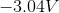Iron (with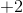oxidation state):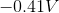Aluminum: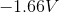Given this information, which of the following redox reactions can be found in a galvanic cell?

I.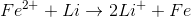II.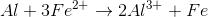III.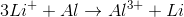III only

I only

I and II

I and III

I and II

Explanation:

Galvanic cells are electrochemical cells that are characterized by a spontaneous redox reaction. To solve this question, we need to find the standard potential for each of the given reactions. Note that each reaction is a redox reaction; therefore, there is an oxidation half-reaction and a reduction half-reaction for each one.

The first reaction has a ferrous ion being reduced (gaining electrons) and lithium being oxidized (losing electrons). From the given information, we can deduce the standard potential for each half-reaction. The standard potential for reduction of iron is. Since the given standard potentials are for "reduction," the oxidation of iron is the opposite of the given value: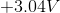. The total standard potential for this reaction is the sum of the standard potential for the half-reaction; therefore, the standard reaction potential for the first reaction is: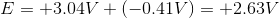Since its standard reaction potential is positive, this reaction is spontaneous and occurs in a galvanic cell.

The second reaction has aluminum being oxidized (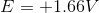) and a ferrous ion being reduced (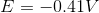). The standard reaction potential is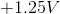; therefore, this reaction can also occur in a galvanic cell.

The third reaction has a lithium ion being reduced (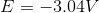) and an aluminum being oxidized (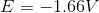). The standard reduction potential is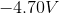; therefore, this reaction is nonspontaneous and cannot occur in a galvanic cell.

### Example Question #171 : Physical Chemistry

What will happen when the two given half-cells are connected by a salt bridge to form a galvanic cell?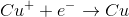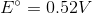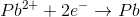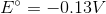Copper metal will be oxidized at the anode; lead ions will be reduced at the cathode.

Lead metal will be oxidized at the anode; copper ions will be reduced at the cathode.

Copper metal will be oxidized at the cathode; lead ions will be reduced at the anode.

No reaction will take place.

Lead metal will be oxidized at the cathode; copper ions will be reduced at the anode.

Lead metal will be oxidized at the anode; copper ions will be reduced at the cathode.

Explanation: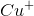has a higher standard reduction potential, and will therefore be reduced, meaning that lead will be oxidized. The anode is where oxidation takes place, and the cathode is where reduction occurs. Therefore, we assign the cathode to copper and the anode to lead.

### Example Question #175 : Physical Chemistry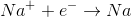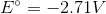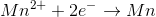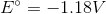For an electrolytic cell containing the two half-cells shown, the __________ reaction will take place and the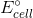will be __________.

nonspontaneous . . .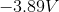nonspontaneous . . .nonspontaneous . . .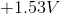spontaneous . . .spontaneous . . .nonspontaneous . . .